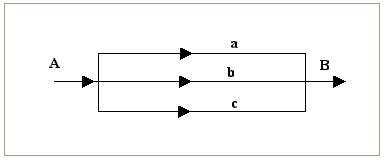### Solving Fluid Mechanics Problems with MS Excel

• Distribution of flow in branches- 28 kB (07-Feb-2001)

For estimating the distribution of flow in branches of pipelines as shown in the diagram:• Pipe friction and losses through fittings- 164 kB (14-Feb-2001)

A 35o API distillate is being transferred from a storage tank at 1 atm absolute pressure to a pressure vessel at 50 psig by means of the piping arrangements shown in figure.The liquid flows at the rate of 23100 lb/hr through 3 inch Schedule 40 steel pipe; the length of the straight pipe is 450 feet. Calculate the minimum horsepower input to the pump having an efficiency of 60 percent.

The properties of the distillate are: viscosity = 3.4 cP, density = 52 lb/ft3.

The following are the data for the pipe and fittings:

For 3 inch Schedule 40 Nominal pipe, OD = 3.5 inch; Thickness = 0.216 inch

Flow coefficients for the fittings (K) are:

Gate valve = 0.25; 90o elbow = 0.9; Check valve = 10

Friction factor can be calculated from Blasius equation. Account for entry and exit losses also.

HOME

Last Modified on: 12-Sep-2014

Chemical Engineering Learning Resources - msubbu
e-mail: msubbu.in[AT]gmail.com
Web: http://www.msubbu.in